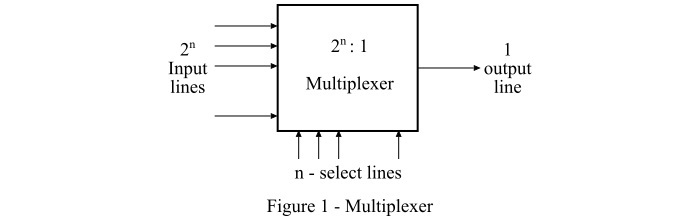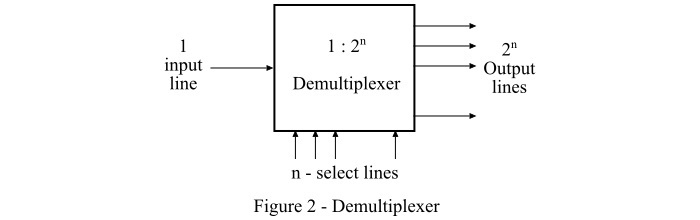# Difference between Multiplexer and Demultiplexer

Both Multiplexer and Demultiplexer are types of combinational digital circuits widely used in many large-scale digital systems. The major difference between the multiplexer and demultiplexer is in their ability to accept multiple input signals and single input signal respectively.

We will explain some other noticeable differences between multiplexer and demultiplexer, but before that have a look on the basic description of multiplexer and demultiplexer which is given in this article.

## What is Multiplexer?

A digital logic circuit which is capable of accepting several inputs and generating a single output is known as multiplexer or MUX. Thus, the multiplexer is a type of data selector which takes many inputs and gives a selected output. In a multiplexer, there are 2n input lines and 1 output line, where n is the number of select lines.

Therefore, a multiplexer is a combinational circuit which is designed to switch one of the many input lines to a single output line by the use of a control signal. For this reason, the multiplexer is also referred to as a many to one circuit. The block diagram of a multiplexer is shown in Figure-1.The multiplexer functions as a multi-position switch which is digitally controlled by a control signal. In case of the multiplexer, the select lines determine that which input signal will get switched to the output line among the many input signals.

## What is Demultiplexer?

A digital combinational circuit which takes one input signal and generates multiple output signals is known as demultiplexer or DEMUX. As it distributes a single input signal over many output lines, hence it is also referred to as a type of data distributor.

In a demultiplexer, there is only 1 input line and 2n output lines. Where, n denotes the number of select lines. Therefore, it can be noted that a demultiplexer reverses the operation of a multiplexer. The block diagram of a demultiplexer is shown in Figure-2.Demultiplexers are used in applications where there is a necessity of connecting single source to several destinations, such as in arithmetical and logic unit, in communication system for data transmission, in wavelength routers, etc.

## Difference between Multiplexer and Demultiplexer

Both multiplexer and demultiplexer are types of combinational digital circuit that are used in several large-scale digital systems. However, there are many differences between a multiplexer and a demultiplexer, which are highlighted in the following table −

Basis of Difference
Multiplexer
Demultiplexer
Definition
A multiplexer is a combinational digital circuit that takes multiple data inputs and provides only single output.A demultiplexer is a combinational digital circuit that takes single input and provides multiple outputs.
Abbreviated name
The abbreviation used to represent the multiplexer is MUX.The abbreviation used to represent the demultiplexer is DEMUX.
Input and output lines
Multiplexer has 2n input lines and 1 output line. Where, n is the number of select lines.Demultiplexer has 1 input line and 2n output lines. Where, n is the number of select lines.
Also known as
Multiplexer is also known as a "data selector".Demultiplexer is also known as "data distributor".
Operating principle
The operating principle of the multiplexer is "many to one".The operating principle of a demultiplexer is "ne to many".
Acts as
Multiplexer acts as a digital multi-position switch.Demultiplexer acts as a digital circuit.
Conversion technique
A multiplexer performs parallel to serial conversion.A demultiplexer performs serial to parallel conversion.
Function of control signal
In case of multiplexer, the function of control signal is to select a specific input that has to be transmitted at the output.In demultiplexer, the function of control signal is to deliver the single input signal over the multiple output lines.
Examples
Examples of some common multiplexers are −
• 8:1 Multiplexer

• 16:1 Multiplexer

• 32:1 Multiplexer

Some common demultiplexers are −
• 1:2 Demultiplexer

• 1:4 Demultiplexer

• 1:8 Demultiplexer

• 1:16 Demultiplexer

Practical importanceIn practice, the multiplexer increases the efficiency of the communication system by enabling the data transmission using a single line.In practice, the demultiplexer takes the output of a multiplexer and convert in its original form at the receiver end.
Usage in time-division multiplexingA multiplexer is used at the transmitter end in the time-division multiplexing (TDM).The demultiplexer is used at the receiver end in the time-division multiplexing.
Applications
The multiplexers are commonly used in communication systems, telephone networks, computer memories, etc.The demultiplexers are used in communication systems, reconstruction of parallel data, ALU, etc.

## Conclusion

Both multiplexers and demultiplexers are required in the communication system because of its bidirectional nature. These two devices perform the exact opposite operations of each other. A major difference between multiplexer and demultiplexer is based on their input and output lines, i.e., a multiplexer has many input lines and one output line, whereas a demultiplexer has one input line and many output lines.

Updated on: 21-Jul-2022

14K+ Views